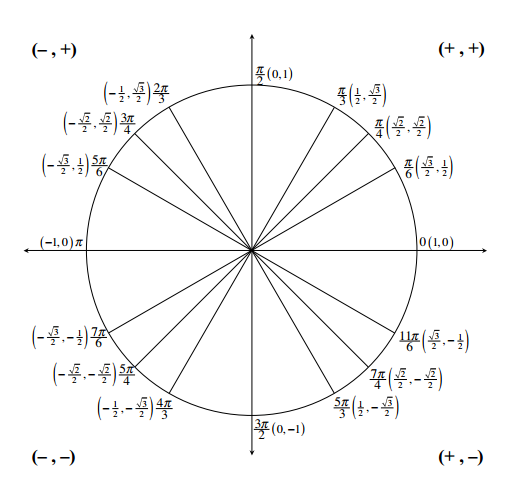### Home > PC3 > Chapter 2 > Lesson 2.3.3 > Problem2-154

2-154.

Evaluate each expression (in radians) and leave your answers in fraction form when appropriate. Remember the restricted ranges for the functions.1. $\sin^{-1}\left(-\frac{\sqrt{3}}{2}\right)$

1. $\sin^{-1}\left(\frac{\sqrt{2}}{2}\right)$

1. $\cos^{-1}\left(-\frac{\sqrt{2}}{2}\right)$

1. $\cos^{-1}\left(\frac{\sqrt{3}}{2}\right)$

The domain of inverse sine is $\left[-\frac{\pi}{2},\frac{\pi}{2}\right]$. The domain of inverse cosine is $[0,\pi]$.